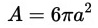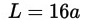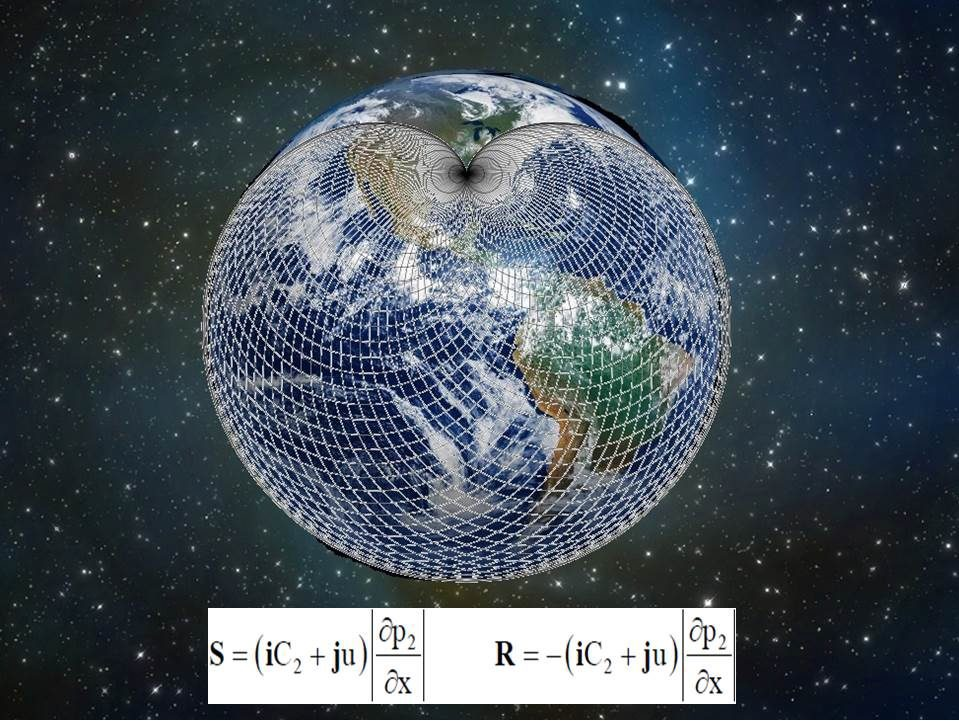# Inertia, 3-D Cardioids and The Topological Metaphor

## Inertia, 3-D Cardioids and The Topological Metaphor

#### Inertia Definition

Inertia describes the relationship between a body and the forces acting upon it, and its motion in response to said forces. The law of inertia states that it is the tendency of an object to resist a change in motion. According to Newton, an object will stay at rest or stay in motion (i.e. 'maintain its velocity' in modern terms) unless acted on by a net external force, whether it results from gravity, friction, contact, or some other source.

#### Newton's Three Laws of Motion

1. An object moves at a constant velocity if there is no net force(or overall force) acting up it to change its speed or direction; objects at rest tend to remain at rest , and object in motion tend to remain in motion with not chain either their speed or the direction. When viewed in an inertial reference frame, an object either remains at rest or continues to move at a constant velocity, unless acted upon by an external force.
2. The vector sum of the forces F on an object is equal to the mass m of that object multiplied by the acceleration vector a of the object; Force = mass x acceleration; or rate of change in momentum; F = ma
3. When one body exerts a force on a second body, the second body simultaneously exerts a force equal in magnitude and opposite in direction on the first body. For any force, there is always an equal and opposite reaction force.

The three laws of motion were first compiled by Isaac Newton in his Philosophiæ Naturalis Principia Mathematica (Mathematical Principles of Natural Philosophy), first published in 1687. Newton used them to explain and investigate the motion of many physical objects and systems. For example, in the third volume of the text, Newton showed that these laws of motion, combined with his law of universal gravitation, explained Kepler's laws of planetary motion.

Netwon’s version of Kepler's third law equates the orbital periods, distance, sand masses of any pair of orbiting objects; ex. the sun and a planet, a planet and a moon , or two stars in a binary star system – see figure; and equation;

#### Conservation Laws in Astronomy

Conservation of Momentum – If there are no external forces, the total momentum of interacting objects cannot change; that is—their total momentum is conserved.

Conservation of Angular Momentum – If there is no external torque, the total angular momentum of a set of interacting objects cannot change; an individual object can change its angular momentum only by transferring some angular momentum to or from another object.

Orbital Angular Momentum – angular momentum = m x v x r; m is earths mass, v is its orbital velocity (the component of velocity perpendicular to r) and r is the radius of the orbit(distance from sum); see figure; because Earth’s angular momentum at any point in its orbit depends on the product of its speed an orbital radius(distance from the sun), earth's orbital speed must be faster when its is nearer to the sun(and the radius is smaller) and slower when it is farther from the sun (and the radius is larger).

Conservation of Energy – Energy is not created or destroyed, it changes forms.

Conservation of Information – Information proceeds through inertial impacts, it changes form, but it is never destroyed.

#### Basic Types of Energy

Kinetic – Energy in motion; Radiative energy – Light energy; can cause changes in materials; Potential energy – Stored Energy; Thermal energy – The total kinetic energy of all of the moving particles in a substance; Gravitational potential energy – The amount of mass and distance to travel before encountering ground; Mass energy – E = mc2; Orbital energy – the sum of a planet’s kinetic and gravitational potential energy; (see figure pg 126)

#### Universal Law of Gravitation (pg 123)

Bounds Orbits – where an object goes around another object multiple times; (pg 124)

Unbound Orbits – paths that bring an object close to another object just once;

Objects Orbiting a Center of Mass – the point at which two objects would balance if they were somehow connected. (see figure pg 125)

Inertial Base Vector

## 3-D Cardioids

A cardioid is a plane curve traced by a point on the perimeter of a circle that is rolling around a fixed circle of the same radius. It is therefore a type of limacon and can be defined as an epicycloid having a single cusp. It is also a type of sinusoidal spiral, and an inverse curve of the parabola with the focus as the center of inversion.

Cardioid Parametric Equations:

The area enclosed by a cardioid can be computed from the polar equation:This is 6 times the area of the circles used in the construction with rolling circles, or 1.5 times the area of the circle used in the construction with circle and tangent lines.

The arc length of a cardioid can be computed exactly, (Rare for algebraic curves. The total length isCausal-Mechanical Equations — The Equations of Time –